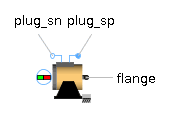Thermal Resistor - MapleSim Help

Thermal Resistor

Lumped thermal element transporting heat without storing itDescription The Thermal Resistor component models a heat transfer element that transports heat without storing it.Equations $\Delta T={T}_{a}-{T}_{b}=R{Q}_{\mathrm{flow}}$ ${Q}_{\mathrm{flow}}={Q}_{{\mathrm{flow}}_{a}}=-{Q}_{{\mathrm{flow}}_{b}}$Variables

 Name Units Description Modelica ID ${Q}_{\mathrm{flow}}$ $W$ Heat flow rate from a to b Q_flow $\mathrm{dT}$ $K$ Temperature difference from a to b dTConnections

 Name Description Modelica ID ${\mathrm{port}}_{a}$ port_a ${\mathrm{port}}_{b}$ port_bParameters

 Name Default Units Description Modelica ID R $\frac{K}{W}$ Constant thermal resistance of material RModelica Standard Library The component described in this topic is from the Modelica Standard Library. To view the original documentation, which includes author and copyright information, click here.